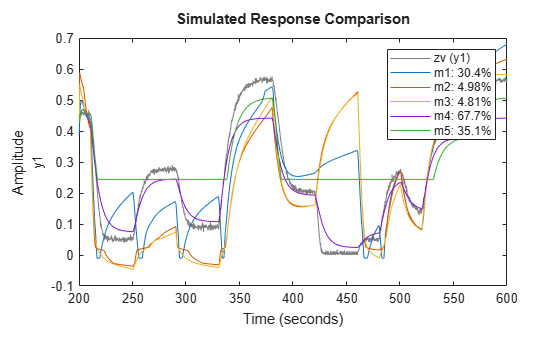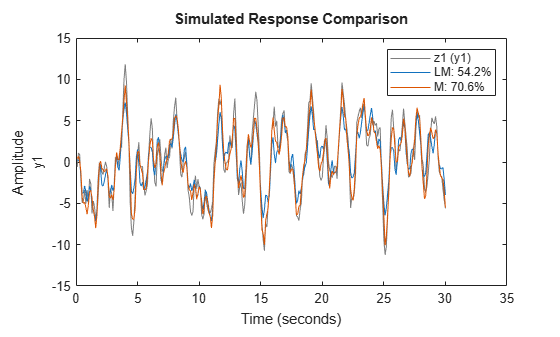## Estimate Hammerstein-Wiener Models at the Command Line

You can estimate Hammerstein-Wiener models after performing the following tasks:

### Estimate Model Using `nlhw`

Use `nlhw` to both construct and estimate a Hammerstein-Wiener model. After each estimation, validate the model by comparing it to other models and simulating or predicting the model response.

Basic Estimation

Start with the simplest estimation using `m = nlhw(data,[nb nf nk])`. For example:

```load iddata3; % nb = nf = 2 and nk = 1 m = nlhw(z3,[2 2 1])```
```m = Hammerstein-Wiener model with 1 output and 1 input Linear transfer function corresponding to the orders nb = 2, nf = 2, nk = 1 Input nonlinearity: Piecewise linear with 10 break-points Output nonlinearity: Piecewise linear with 10 break-points Sample time: 1 seconds Status: Termination condition: Maximum number of iterations reached.. Number of iterations: 20, Number of function evaluations: 185 Estimated using NLHW on time domain data "z3". Fit to estimation data: 75.31% FPE: 2.019, MSE: 1.472 More information in model's "Report" property. ```

The second input argument [`nb` `nf` `nk`] sets the order of the linear transfer function, where `nb` is the number of zeros plus 1, `nf` is the number of poles, and `nk` is the input delay. By default, both the input and output nonlinearity estimators are piecewise linear functions (see the `idPiecewiseLinear` reference page). `m` is an `idnlhw` object.

For MIMO systems, `nb`, `nf`, and `nk` are ny-by-nu matrices. See the `nlhw` reference page for more information about MIMO estimation.

### Configure Nonlinearity Estimators

You can specify a different nonlinearity estimator than the default piecewise linear estimators.

`m = nlhw(data,[nb,nf,nk],InputNL,OutputNL)`

`InputNL` and `OutputNL` are nonlinearity estimator objects. If your input signal is binary, set `InputNL` to `idUnitGain`.

To use nonlinearity estimators with default settings, specify `InputNL` and `OutputNL` using constructors with no input arguments or their names as character vectors (such as 'idWaveletNetwork' for wavelet network or 'idSigmoidNetwork' for sigmoid network).

```load iddata3; m = nlhw(z3,[2 2 1],'idSigmoidNetwork','idDeadZone');```

If you need to configure the properties of a nonlinearity estimator, use its object representation. For example, to estimate a Hammerstein-Wiener model that uses saturation as its input nonlinearity and one-dimensional polynomial of degree 3 as its output nonlinearity:

`m = nlhw(z3,[2 2 1],'idSaturation',idPolynomial1D(3));`

The third input `'idSaturation'` specifies the saturation nonlinearity with default property values. `idPolynomial1D(3)` creates a one-dimensional polynomial object of degree 3. Of course, you could have used the constructor idSaturation directly in place of the character vector 'idSaturation'.

For MIMO models, specify the nonlinearities using objects, or cell array of character vectors representing the nonlinearity names. If single name (character vector) used, the same value is applied to all the channels.

This table summarizes values that specify the nonlinearity estimators.

NonlinearityValue (Default Nonlinearity Configuration)Class
Piecewise linear
(default)
`'idPiecewiseLinear'` `idPiecewiseLinear`
One layer sigmoid network`'idSigmoidNetwork'` `idSigmoidNetwork`
Wavelet network`'idWaveletNetwork'` `idWaveletNetwork`
Saturation`'idSaturation'` `idSaturation`
Dead zone`'idDeadZone'` `idDeadZone`
One-
dimensional polynomial
`'idPolynomial1D'` `idPolynomial1D`
Unit gain`'idUnitGain'` or `[ ]``idUnitGain`
Neural Network`'idNeuralNetwork'``idNeuralNetwork`

Additional available nonlinearities include custom networks that you create. Specify a custom network by defining a function called `gaussunit.m`, as described in the `idCustomNetwork` reference page. Define the custom network object `CNetw` as:

```CNetw = idCustomNetwork(@gaussunit); m = nlhw(z3,[2 2 1],'idSaturation',CNetw);```

### Exclude Input or Output Nonlinearity

Exclude a nonlinearity for a specific channel by specifying the `idUnitGain` value for the `InputNonlinearity` or `OutputNonlinearity` properties.

If the input signal is binary, set `InputNL` to `idUnitGain`.

For more information about model estimation and properties, see the `nlhw` and `idnlhw` reference pages.

For a description of each nonlinearity estimator, see Available Nonlinearity Estimators for Hammerstein-Wiener Models.

### Iteratively Refine Model

Estimate a Hammerstein-Wiener model and then use `nlhw` command to iteratively refine the model.

```load iddata3; m1 = nlhw(z3,[2 2 1],idSigmoidNetwork, idWaveletNetwork); m2 = nlhw(z3,m1);```

Alternatively, use `pem` to refine the model.

`m2 = pem(z3,m1);`

Check the search termination criterion in `m.Report.Termination.WhyStop`. If `WhyStop` indicates that the estimation reached the maximum number of iterations, try repeating the estimation and possibly specifying a larger value for the `MaxIterations`.

Run 30 more iterations starting at model `m1`.

```opt = nlhwOptions; opt.SearchOptions.MaxIterations = 30; m2 = nlhw(z3,m1,opt);```

When the `m.Report.Termination.WhyStop` value is `Near (local) minimum, (norm(g) < tol` or `No improvement along the search direction with line search`, validate your model to see if this model adequately fits the data. If not, the solution might be stuck in a local minimum of the cost-function surface. Try adjusting the `SearchOptions.Tolerance` or the `SearchMethod` option of the `nlhw` option set, and repeat the estimation.

You can also try perturbing the parameters of the last model using `init`, and then refine the model using `nlhw` command.

Randomly perturb parameters of original model `m1` about nominal values.

`m1p = init(m1);`

Estimate the parameters of perturbed model.

`M2 = nlhw(z3,m1p);`

Note that using `init` does not guarantee a better solution on further refinement.

### Improve Estimation Results Using Initial States

If your estimated Hammerstein-Wiener model provides a poor fit to measured data, you can repeat the estimation using the initial state values estimated from the data. By default, the initial states corresponding to the linear block of the Hammerstein-Wiener model are zero.

To specify estimating initial states during model estimation:

```load iddata3; opt = nlhwOptions('InitialCondition', 'estimate'); m = nlhw(z3,[2 2 1],idSigmoidNetwork,[],opt);```

### Troubleshoot Estimation

If you do not get a satisfactory model after many trials with various model structures and estimation options, it is possible that the data is poor. For example, your data might be missing important input or output variables and does not sufficiently cover all the operating points of the system.

Nonlinear black-box system identification usually requires more data than linear model identification to gain enough information about the system. See also Troubleshooting Model Estimation.

### Estimate Multiple Hammerstein-Wiener Models

This example shows how to estimate and compare multiple Hammerstein-Wiener models using measured input-output data.

```load twotankdata z = iddata(y,u,0.2); ze = z(1:1000); zv = z(1001:3000);```

Estimate several models using the estimation data `ze` and different model orders, delays, and nonlinearity settings.

```m1 = nlhw(ze,[2 3 1]); m2 = nlhw(ze,[2 2 3]); m3 = nlhw(ze,[2 2 3],idPiecewiseLinear('NumberofUnits',13),idPiecewiseLinear('NumberofUnits',10)); m4 = nlhw(ze,[2 2 3],idSigmoidNetwork('NumberofUnits',2),idPiecewiseLinear('NumberofUnits',10));```

An alternative way to perform the estimation is to configure the model structure first using `idnlhw`, and then estimate the model.

```m5 = idnlhw([2 2 3],idDeadZone,idSaturation); m5 = nlhw(ze,m5);```

Compare the resulting models by plotting the model outputs and the measured output in validation data `zv`.

`compare(zv,m1,m2,m3,m4,m5)`### Improve a Linear Model Using Hammerstein-Wiener Structure

This example shows how to use the Hammerstein-Wiener model structure to improve a previously estimated linear model.

After estimating the linear model, insert it into the Hammerstein-Wiener structure that includes input or output nonlinearities.

Estimate a linear model.

```load iddata1 LM = arx(z1,[2 2 1]);```

Extract the transfer function coefficients from the linear model.

`[Num,Den] = tfdata(LM);`

Create a Hammerstein-Wiener model, where you initialize the linear block properties `B` and `F` using `Num` and `Den`, respectively.

```nb = 1; % In general, nb = ones(ny,nu) % ny is number of outputs and nu is number of inputs nf = nb; nk = 0; % In general, nk = zeros(ny,nu) % ny is number of outputs and nu is number of inputs M = idnlhw([nb nf nk],[],'idPiecewiseLinear'); M.B = Num; M.F = Den;```

Estimate the model coefficients, which refines the linear model coefficients in `Num` and `Den` .

`M = nlhw(z1,M);`

Compare responses of linear and nonlinear model against measured data.

`compare(z1,LM,M);`